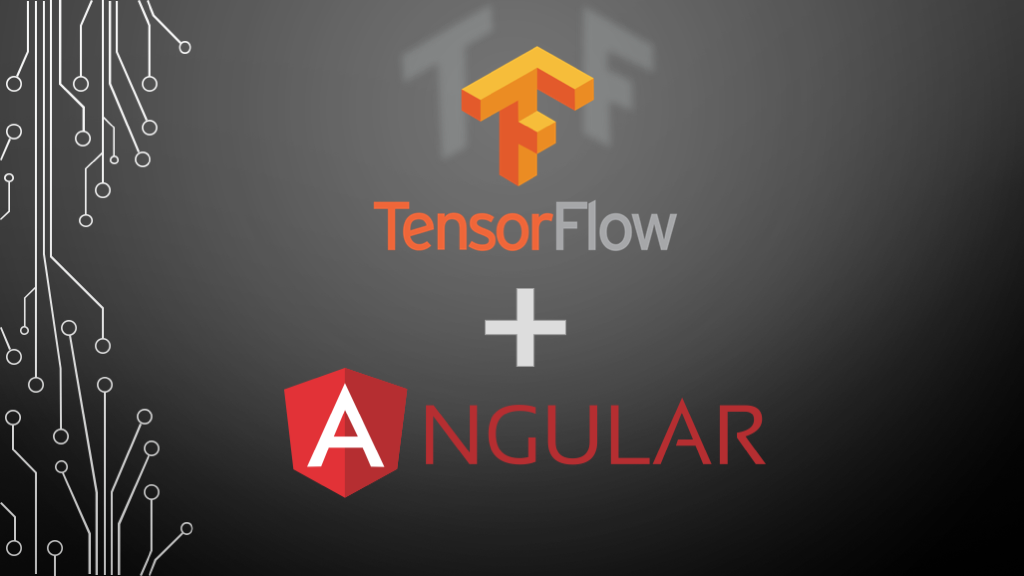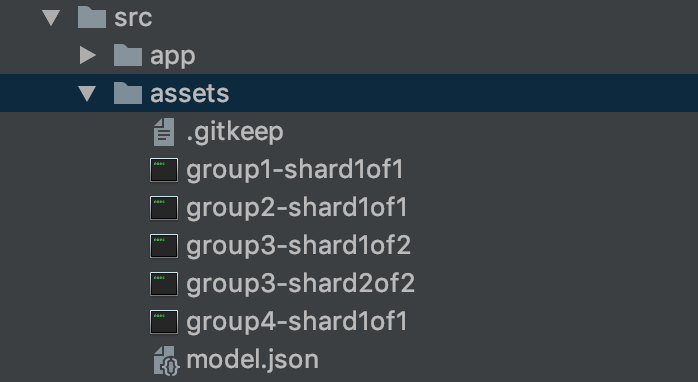Goalist Developers Blog

# Using pre-trained Machine Learning (ML) Models in the browser with TensorFlow.js & Angular

Greetings for the day! My name is Vivek.

In this blog post, let's see how to use your pre-trained Machine Learning (ML) model directly in the browser using Tensorflow.js and AngularThe following section of this blog is interactive, so you can try to draw a number between 0 ~ 9 and see the predicted output in the browser⤵︎
Go ahead and try it yourself, draw a number inside this blue box↓

Amazzing isn't it? Let's learn how to do this step by step

### #Step 1) Convert your Keras model to load into TensorFlow.js

TensorFlow for Javascript has a Python CLI tool that converts an h5 model saved in Keras to a set of files that can be used on the web.
To install it, run the following command

`pip install tensorflowjs`

At this point, you will need to have a Keras model saved on your local system.

Suppose you have your Keras Model save at the following location
input_path/file_name.h5
and suppose you want to generate output at the following location
path_to_output_folder/
In that case your command to convert model will look something like this

```tensorflowjs_converter --input_format keras \
input_path/file_name.h5 \
path_to_output_folder
```

In my case, the model is located in keras/cnn.h5 and I would like to keep my converted model at src/assets directory so I shall run the following command

```tensorflowjs_converter --input_format keras \
keras/cnn.h5 \
src/assets
```

Input and Output directories should look similar to this
InputOutput### #Step 2) Load the converted model into your Angular component

To load the model, you need TensorFlow.js library in your Angular application
Install it using Node Package Manager

`npm install @tensorflow/tfjs --save`

Here is how to load the model into your component

```import {Component, OnInit} from '@angular/core';
import * as tf from '@tensorflow/tfjs';

@Component({
selector: 'app-root',
templateUrl: './app.component.html',
styleUrls: ['./app.component.scss'],
})
export class AppComponent implements OnInit {

model: tf.Model;

ngOnInit() {
this.loadModel();
}

// Load pre-trained KERAS model
async loadModel() {
this.model = await tf.loadModel('./assets/model.json');
}

}
```

### #Step 3) Make predictions using live drawn image data in the browser

Now that our model is loaded, it is expecting 4-dimensional image data in a shape of
[any, 28, 28, 1]
[batchsize, width pixels, height pixels, color channels]

Just trying to avoid memory leaks and to clean up the intermediate memory allocated to the tensors we run our predictions inside of `tf.tidy()` ( TensorFlow.js)

TensorFlow.js gives us a fromPixels (TensorFlow.js) helper to convert an ImageData HTML object into a Tensor.
So the complete code looks like this ↓

```import {Component, OnInit} from '@angular/core';

import * as tf from '@tensorflow/tfjs';

@Component({
selector: 'app-root',
templateUrl: './app.component.html',
styleUrls: ['./app.component.scss'],
})
export class AppComponent implements OnInit {

model: tf.Model;
predictions: any;

ngOnInit() {
this.loadModel();
}

// Load pretrained KERAS model
async loadModel() {
this.model = await tf.loadModel('./assets/model.json');
}

// Do predictions
async predict(imageData: ImageData) {

const pred = await tf.tidy(() => {

// Convert the canvas pixels to
let img = tf.fromPixels(imageData, 1);
// @ts-ignore
img = img.reshape([1, 28, 28, 1]);
img = tf.cast(img, 'float32');

// Make and format the predications
const output = this.model.predict(img) as any;

// Save predictions on the component
this.predictions = Array.from(output.dataSync());
});

}

}
```

And component HTML looks like this

```<div class="container">

<!--Input Section-->
<div class="column justify-content-center">
<div class="col-sm">
<h5>Draw a number here </h5>
<div class="wrapper">
<canvas drawable (newImage)="predict(\$event)"></canvas>
<br>
</div>
<button class="btn btn-sm btn-warning" (click)="canvas.clear()">Erase</button>
</div>

<!--Prediction Section-->
<div class="col-sm predict">
<h5>TensorFlow Prediction</h5>
<chart [data]="predictions"></chart>
</div>
</div>
</div>

<router-outlet></router-outlet>
```

There we go... we just used Machine Learning in the browser.

Learn more about using TensorFlow.js here youtu.be

To learn more about the methods used in the tutorial refer to this js.tensorflow.org

That's all for now, see you next time with some more TensorFlow stuff... till then
Happy Learning !!